Next: 3.1.4 Lattice Heat Flow Up: 3.1 The Device Simulator Previous: 3.1.2 Drift-Diffusion Current Relations

## 3.1.3 Hydrodynamic Model Current Equations

For the hydrodynamic (HD) transport model used in this work the current densities are obtained for electrons as: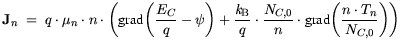(3.6)

and for holes:(3.7)

Comparing to the DD approach the carrier temperature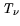can deviate from the lattice temperature. The conservation of the average energy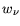is written:
 div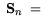grad(3.8)

 div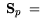grad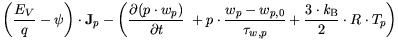(3.9)

The energy fluxes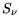are defined as: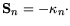grad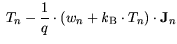(3.10)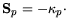grad(3.11)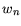and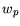denote the average carrier energies,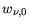the equilibrium energies. Theare approximated as: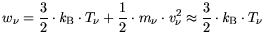(3.12)

The first term represents the carrier thermal energy while the second denotes the carrier kinetic energy. In this work the carrier kinetic energy is neglected against the thermal energy. Some publications name the simplified transport model an energy transport model. The impact of this simplification, which goes along with a limited number of moments considered for the solution of the Boltzmann equation, and the results for device application from both numerical and accuracy aspects are discussed in Section 3.6. The carrier thermal conductivities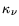are defined for electrons: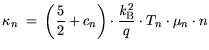(3.13)

and for holes: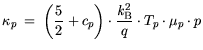(3.14)

(3.14) and (3.15) represent a generalized Wiedemann-Franz law. The heat capacities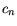and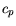of the electron and hole gases are typically neglected.Next: 3.1.4 Lattice Heat Flow Up: 3.1 The Device Simulator Previous: 3.1.2 Drift-Diffusion Current Relations
Quay
2001-12-21